# Exercises¶

## Day 1¶

1. Consider a reduced density matrix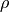corresponding to a maximally mixed state in a Hilbert space of dimension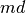. Compute the truncation error associated with keeping only the largest m eigenvectors of. Fortunately, the reduced density matrix eigenvalues for ground states of local Hamiltonians decay much more quickly!

2. Explore computing the ground state energy of the Heisenberg model using the infinite system algorithm. The exact Bethe ansatz result in the thermodynamic limit is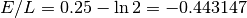. Note the respectable accuracy obtained with an extremely small block basis of size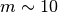. Why does the DMRG work so well in this case?

3. Entanglement entropy:

1. Calculate the bipartite (von Neumann) entanglement entropy at the center of the chain during the infinite system algorithm. How does it scale with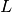?

2. Now, using the finite system algorithm, calculate the bipartite entanglement entropy for every bipartite splitting. How does it scale with subsystem size?

Hint

To create a simple plot in python:

>>> from matplotlib import pyplot as plt
>>> x_values = [1, 2, 3, 4]
>>> y_values = [4, 2, 7, 3]
>>> plt.plot(x_values, y_values)
>>> plt.show()

3. From the above, estimate the central chargeof the “Bethe phase” (1D quasi-long-range Néel phase) of the 1D Heisenberg model, and in light of that, think again about your answer to the last part of exercise 2.

The formula for fitting the central charge on a system with open boundary conditions is: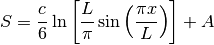where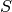is the von Neumann entropy.

Hint

To fit a line in python:

>>> x_values = [1, 2, 3, 4]
>>> y_values = [-4, -2, 0, 2]
>>> slope, y_intercept = np.polyfit(x_values, y_values, 1)

4. XXZ model:

1. Change the code (ever so slightly) to accommodate spin-exchange anisotropy: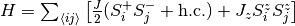.
2. For(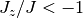), the ground state is known to be an Ising antiferromagnet (ferromagnet), and thus fully gapped. Verify this by investigating scaling of the entanglement entropy as in exercise 3. What do we expect for the central charge in this case?

## Day 2¶

1. Using simple_dmrg_03_conserved_quantum_numbers.py, calculate the “spin gap”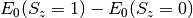. How does the gap scale with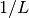? Think about how you would go about computing the spectral gap in the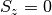sector: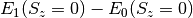, i.e., the gap between the ground state and first excited state within thesector.

2. Calculate the total weight of each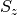sector in the enlarged system block after constructing each block of. At this point, it’s important to fully understand whyis indeed block diagonal, with blocks labeled by the total quantum numberfor the enlarged system block.

3. Starting with simple_dmrg_02_finite_system.py, implement a spin-spin correlation function measurement of the free two sites at each step in the finite system algorithm, i.e., calculate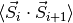for all. In exercise 3 of yesterday’s tutorial, you should have noticed a strong period-2 oscillatory component of the entanglement entropy. With your measurement of, can you now explain this on physical grounds?

Answer: finite_system_algorithm(L=20, m_warmup=10, m_sweep_list=[10, 20, 30, 40, 40]) with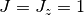should give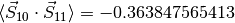on the last step.

4. Implement the “ring term”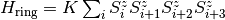. Note that this term is one of the pieces of the SU(2)-invariant four-site ring-exchange operator for sites (,,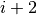,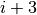), a term which is known to drive the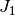-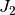Heisenberg model on the two-leg triangular strip into a quasi-1D descendant of the spinon Fermi sea (“spin Bose metal”) spin liquid [see http://arxiv.org/abs/0902.4210].

Answer: finite_system_algorithm(L=20, m_warmup=10, m_sweep_list=[10, 20, 30, 40, 40]) with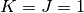, should give.